We're building the future of Supply Chain Security. View Roles

## Risk Ledger Blog

The latest updates, stories, ideas and guides from the Risk Ledger team.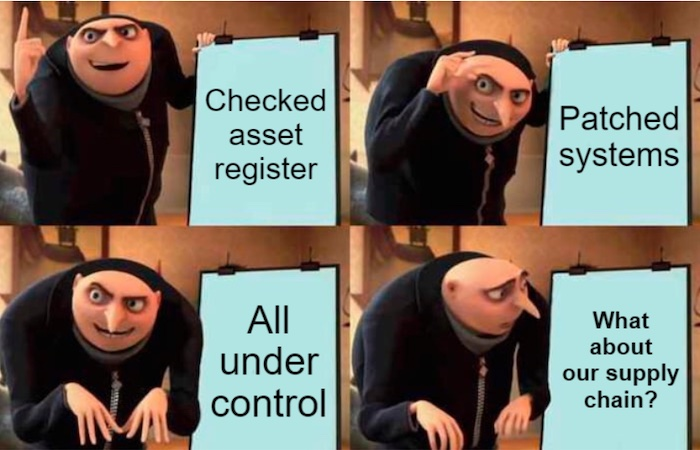Risk LedgerRisk Ledger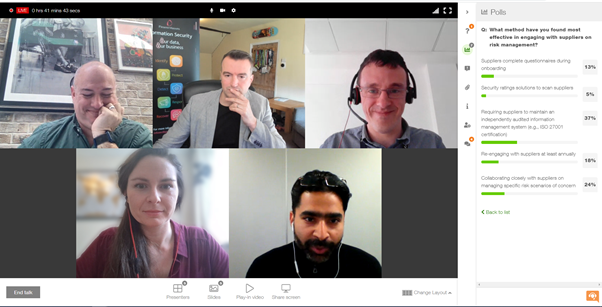Emily HodgesEmily Hodges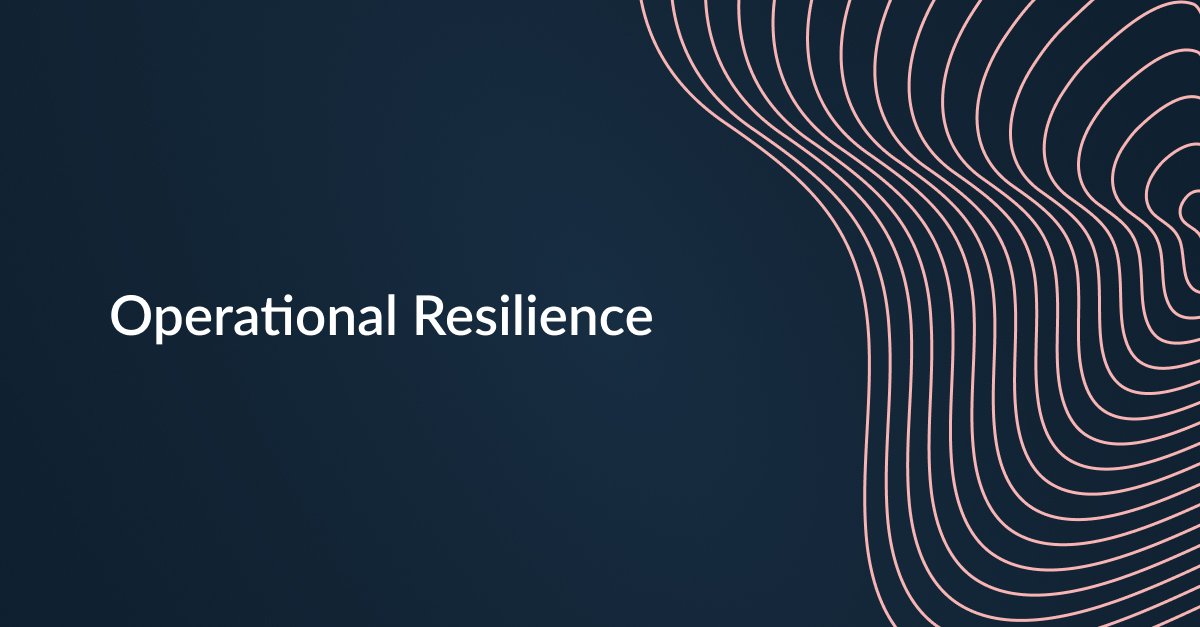Risk LedgerRisk Ledger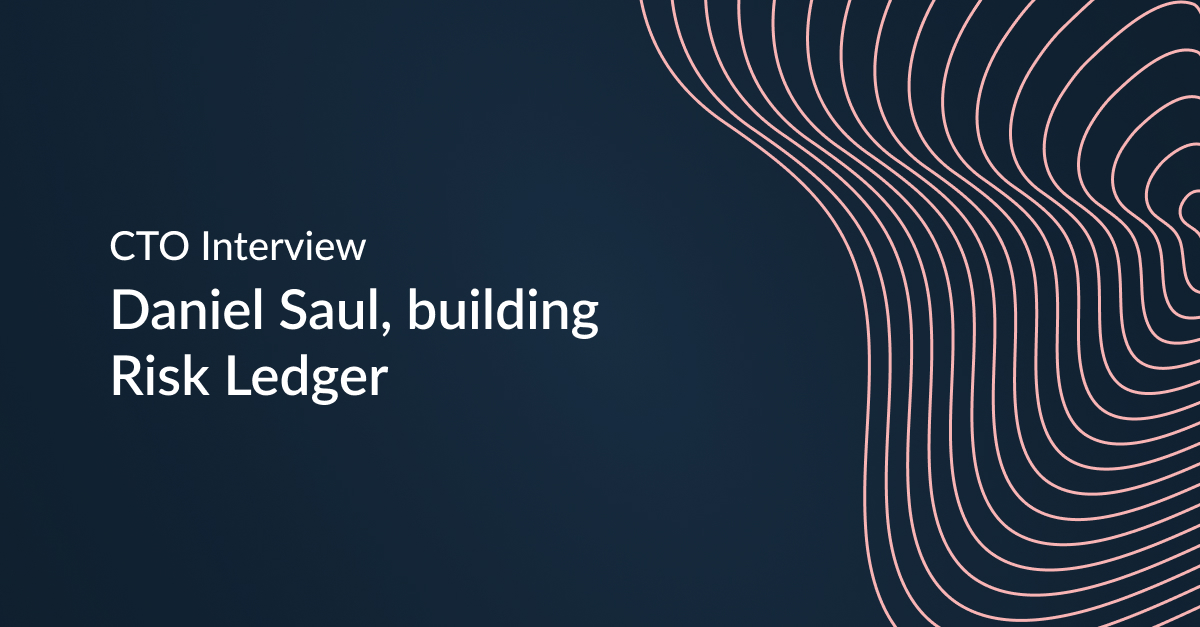Risk LedgerRisk Ledger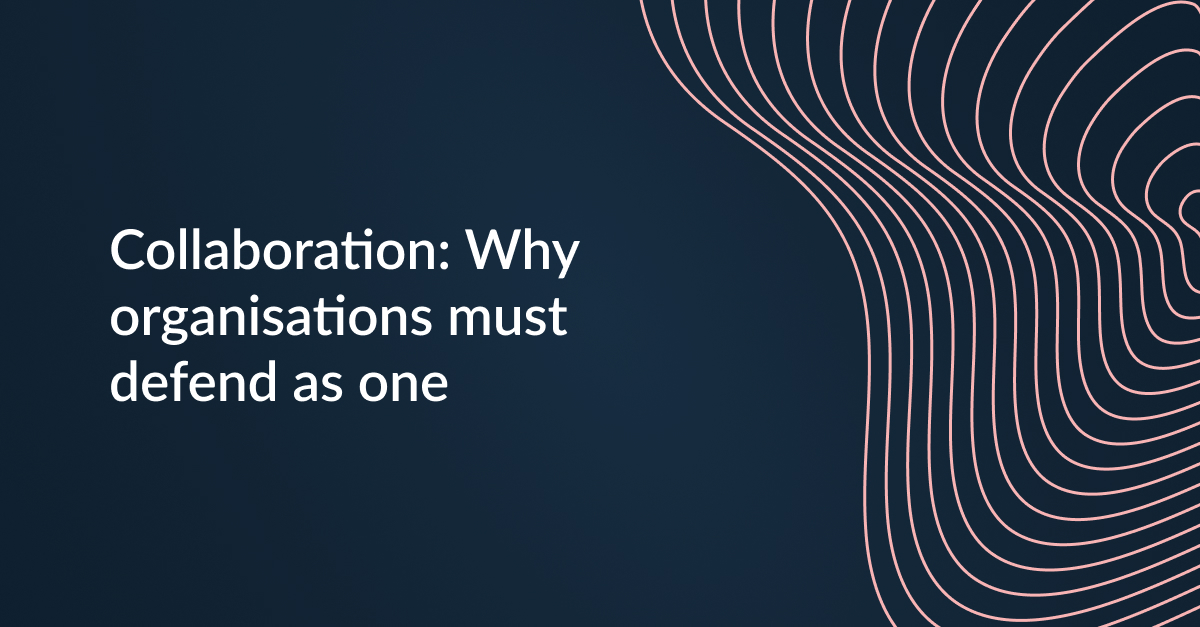Risk LedgerRisk Ledger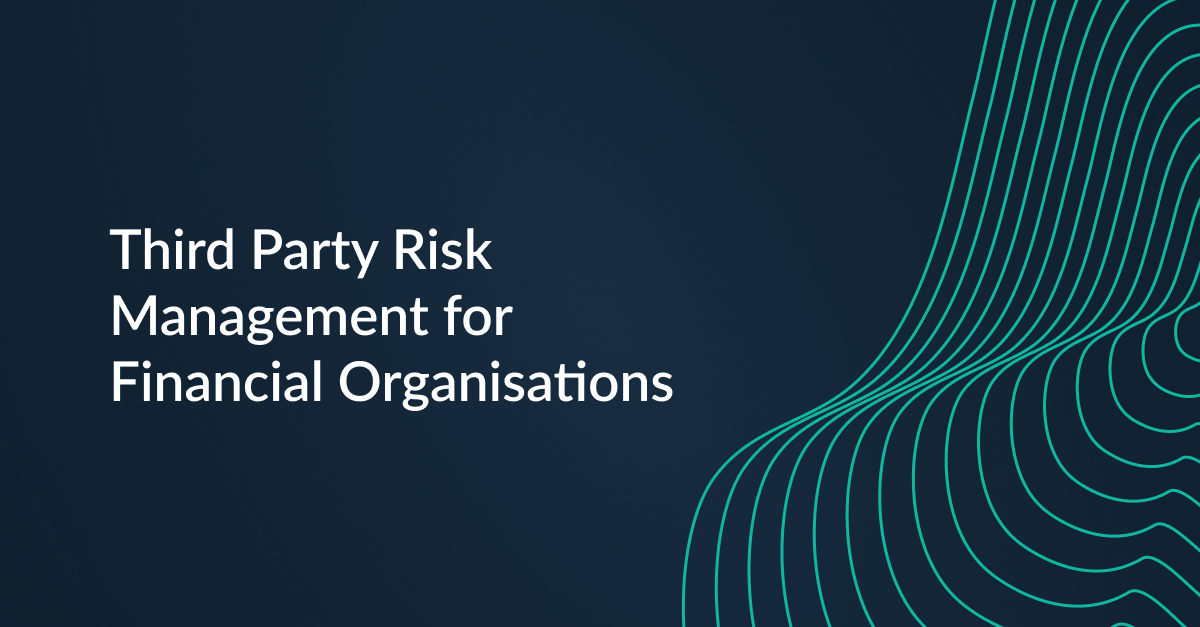Risk LedgerRisk Ledger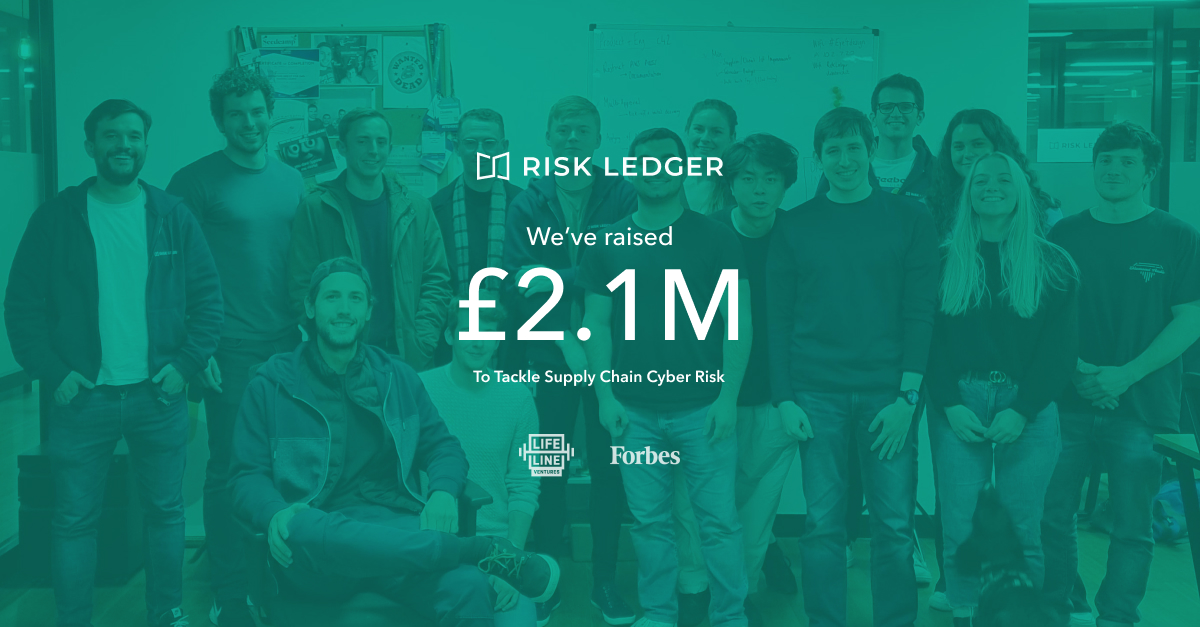Risk LedgerRisk Ledger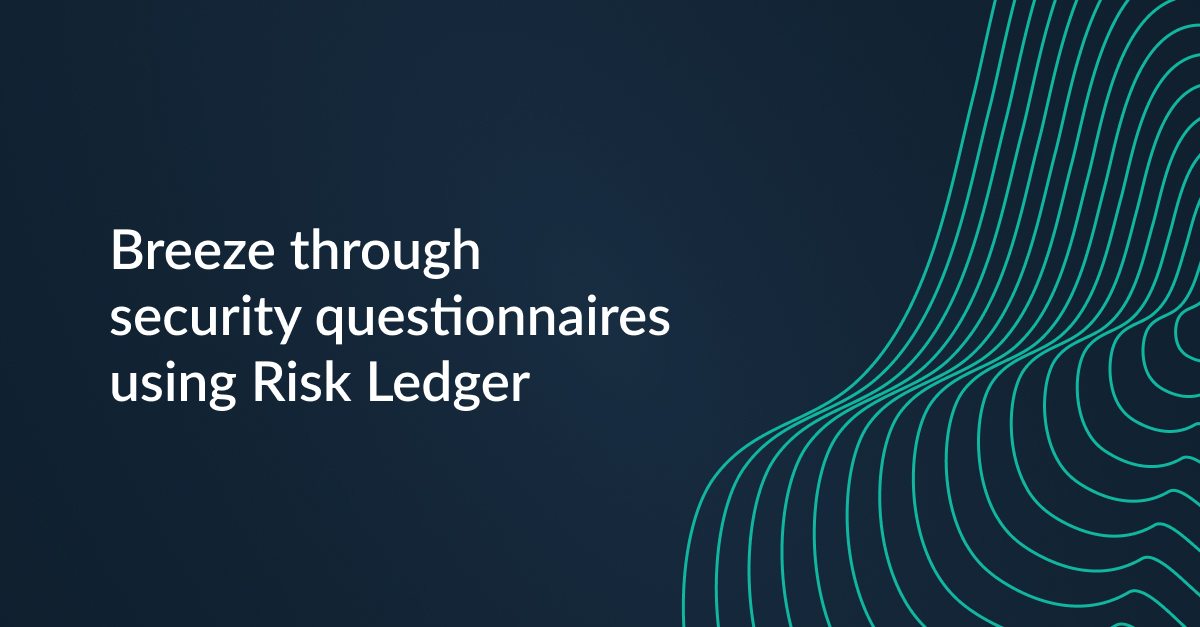Laura WhiteLaura White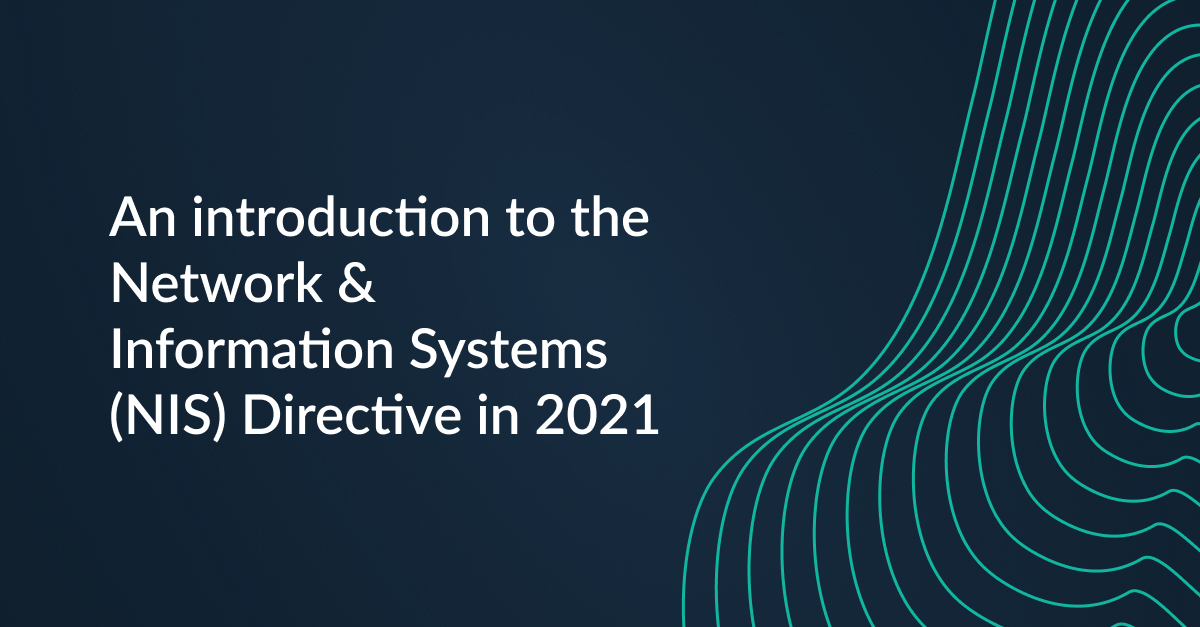Risk LedgerRisk Ledger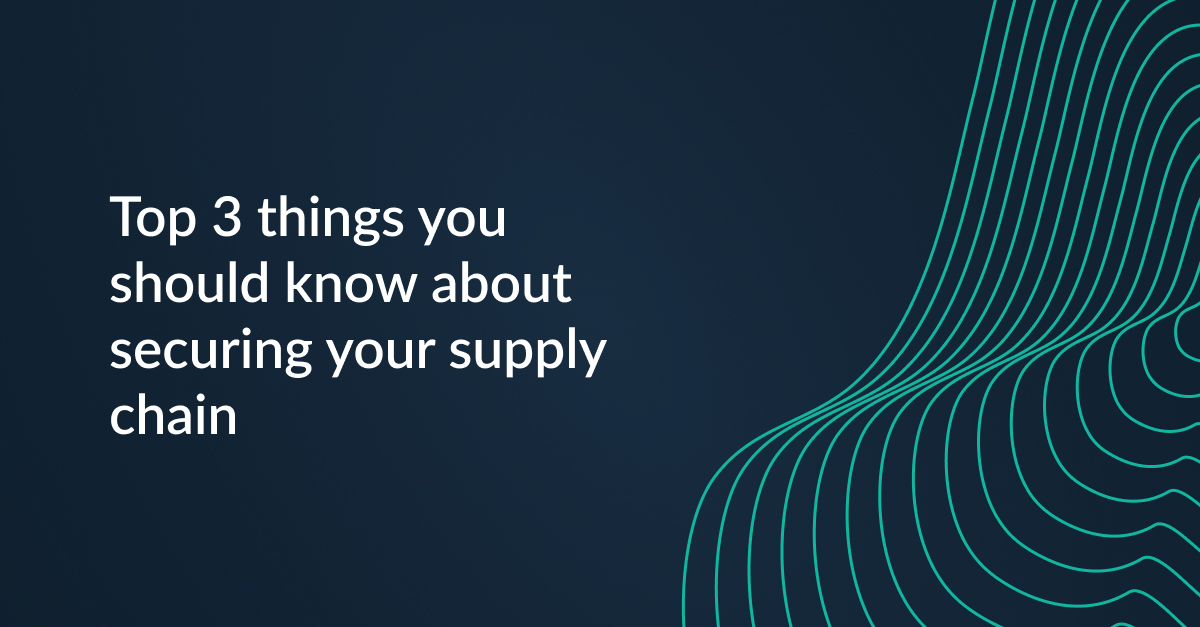Risk LedgerRisk Ledger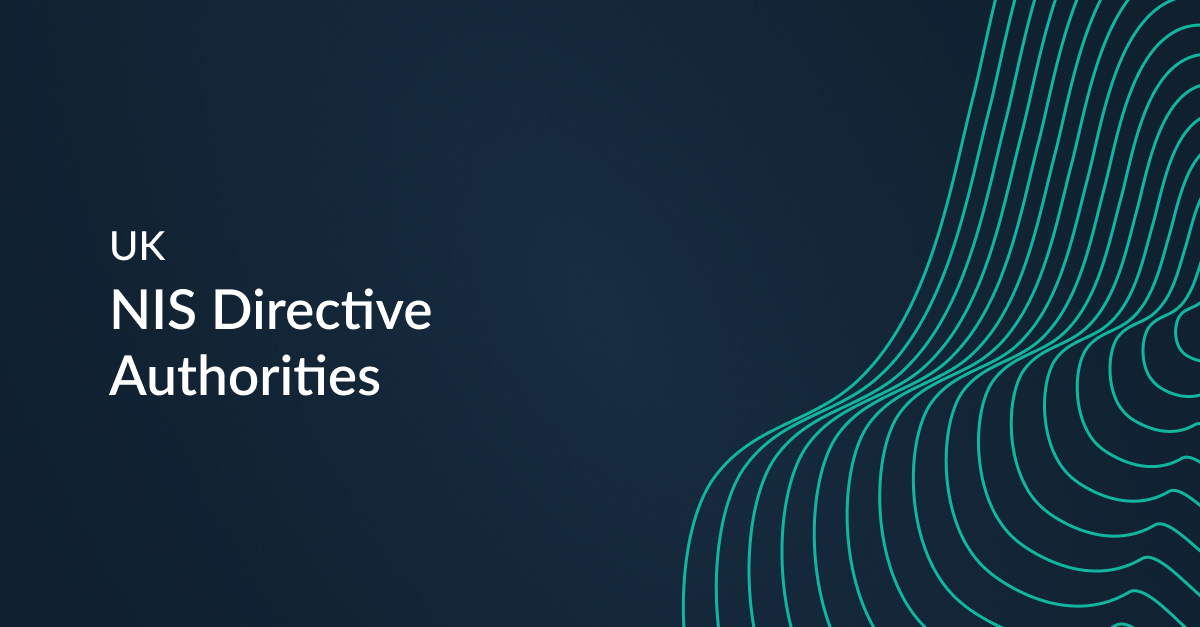Risk LedgerRisk Ledger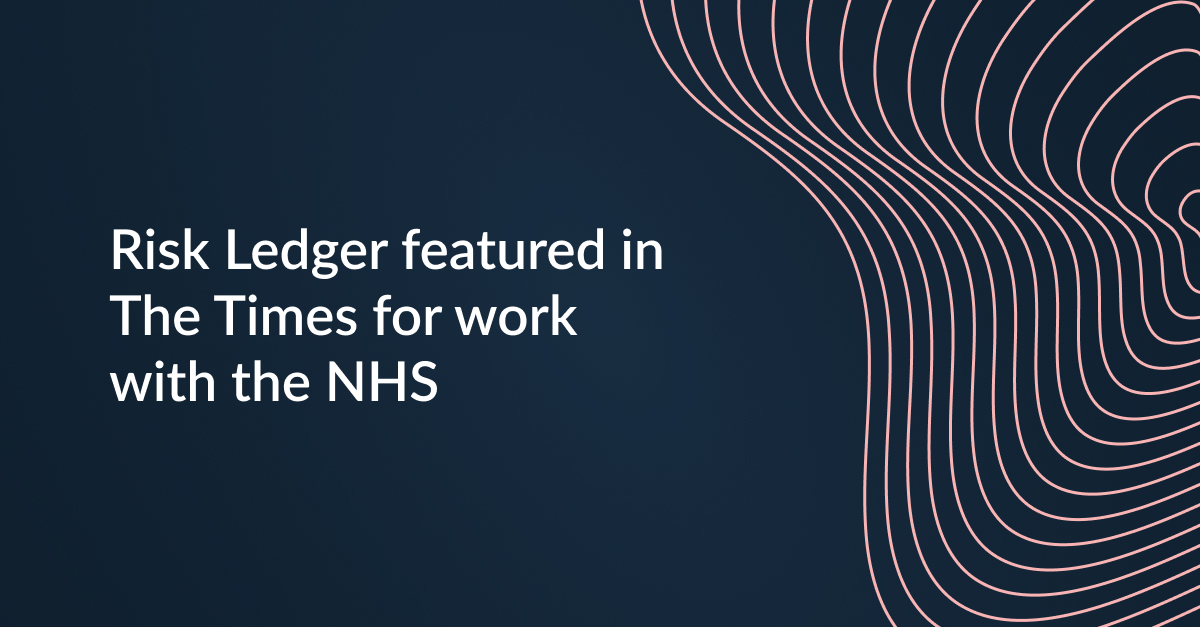Risk LedgerRisk Ledger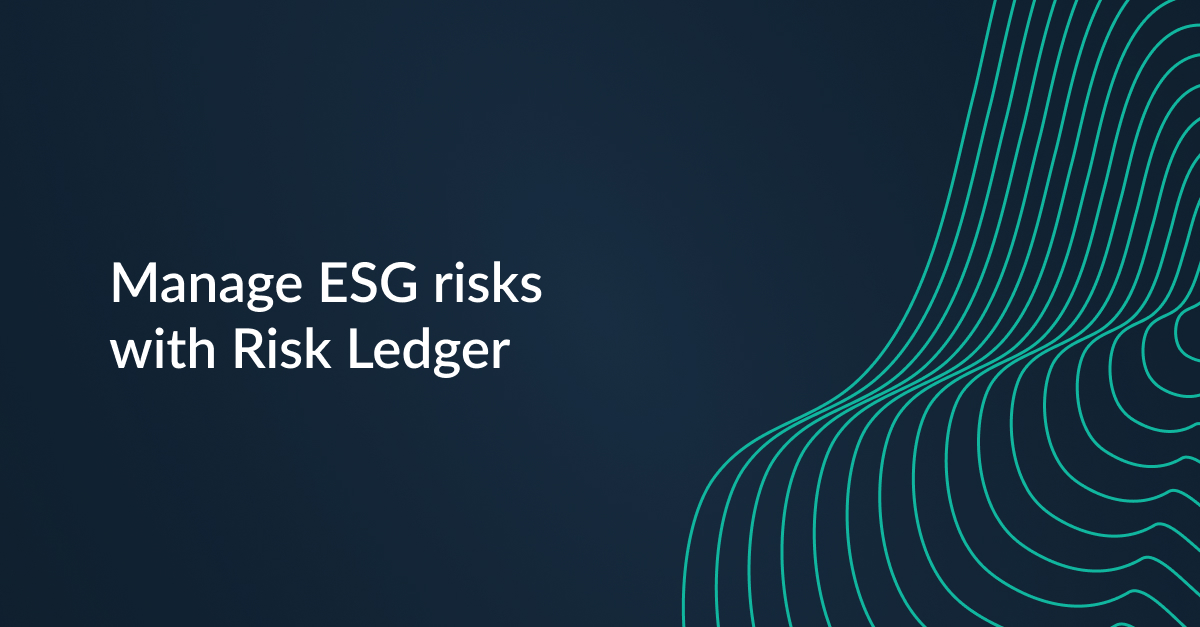Risk LedgerRisk Ledger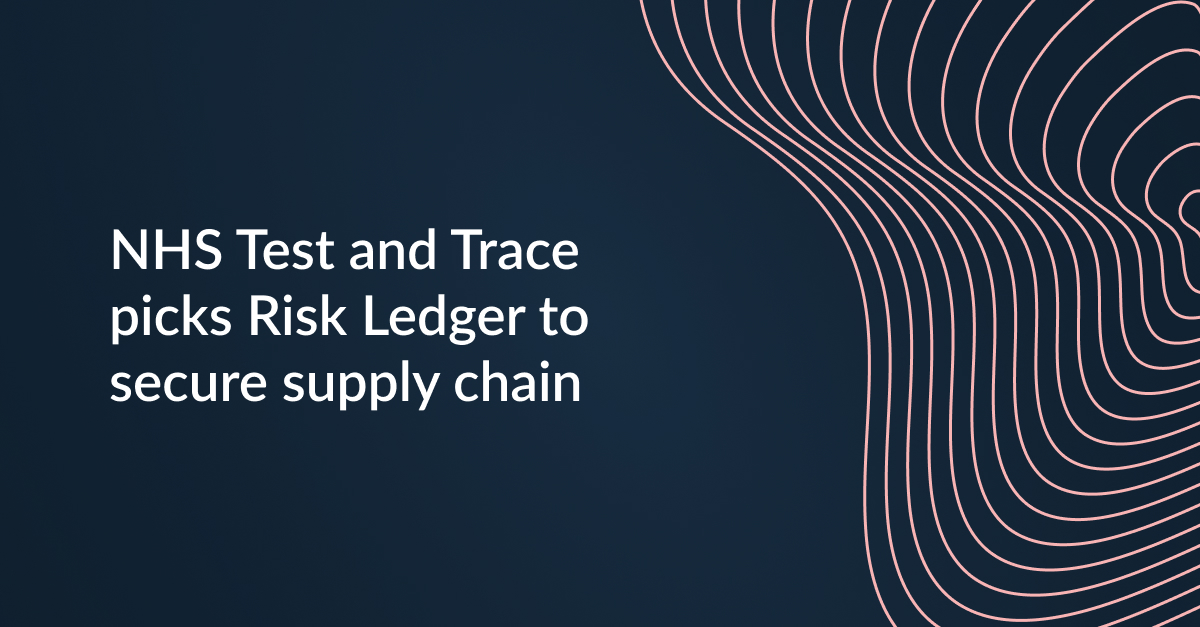Risk LedgerRisk LedgerRisk LedgerRisk LedgerRisk LedgerRisk Ledger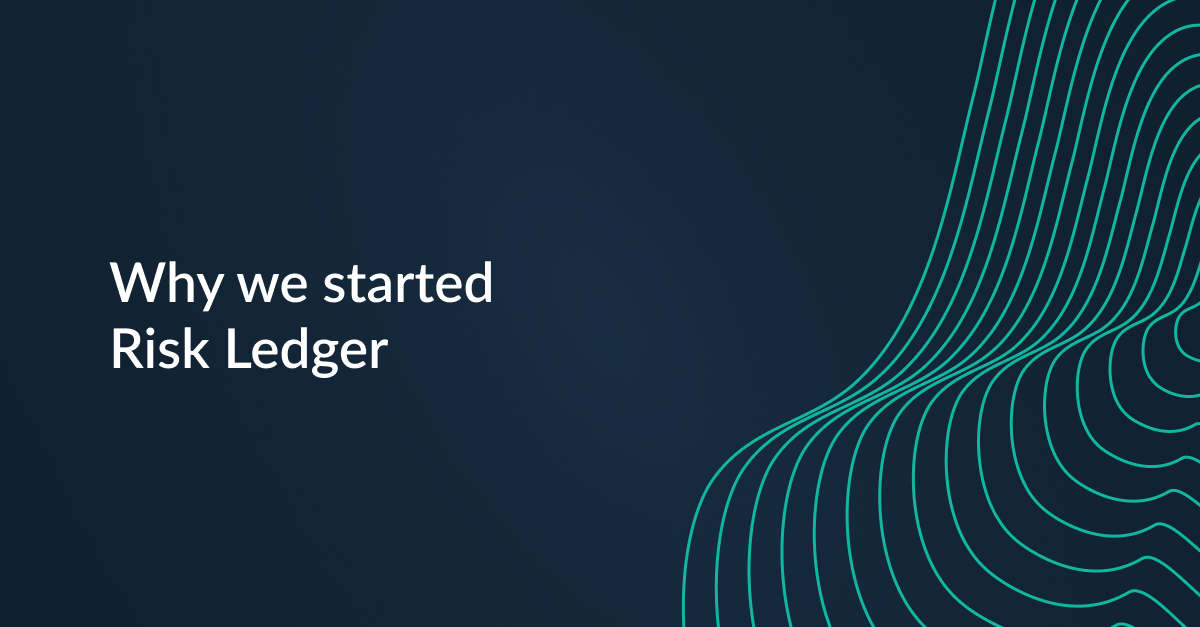Risk LedgerRisk Ledger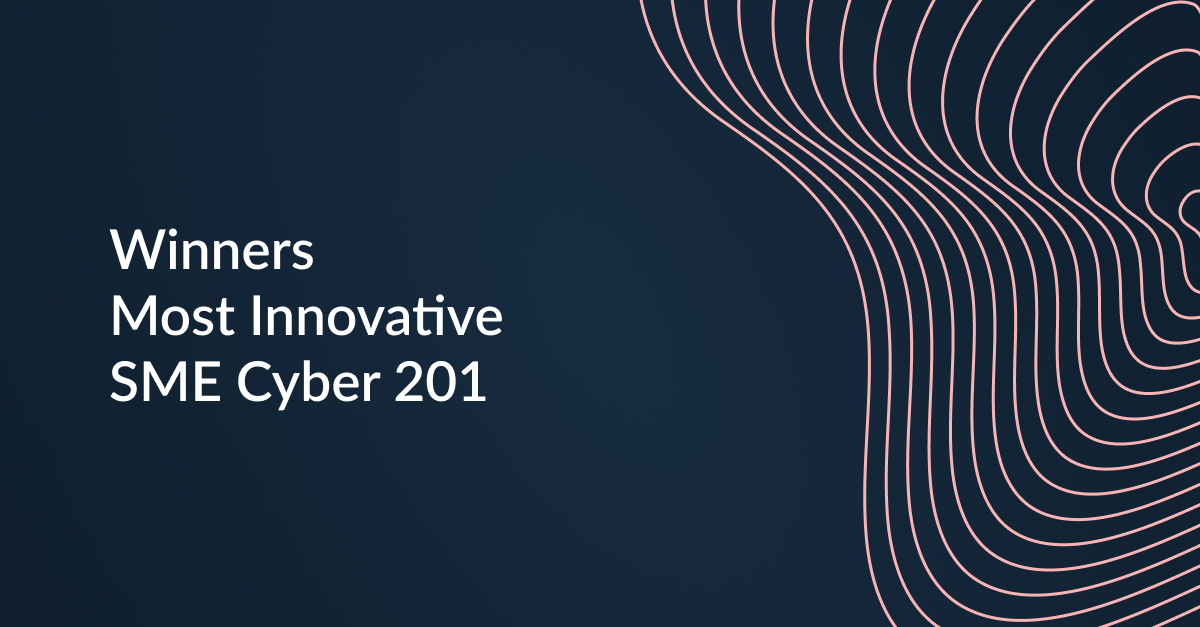Risk LedgerRisk Ledger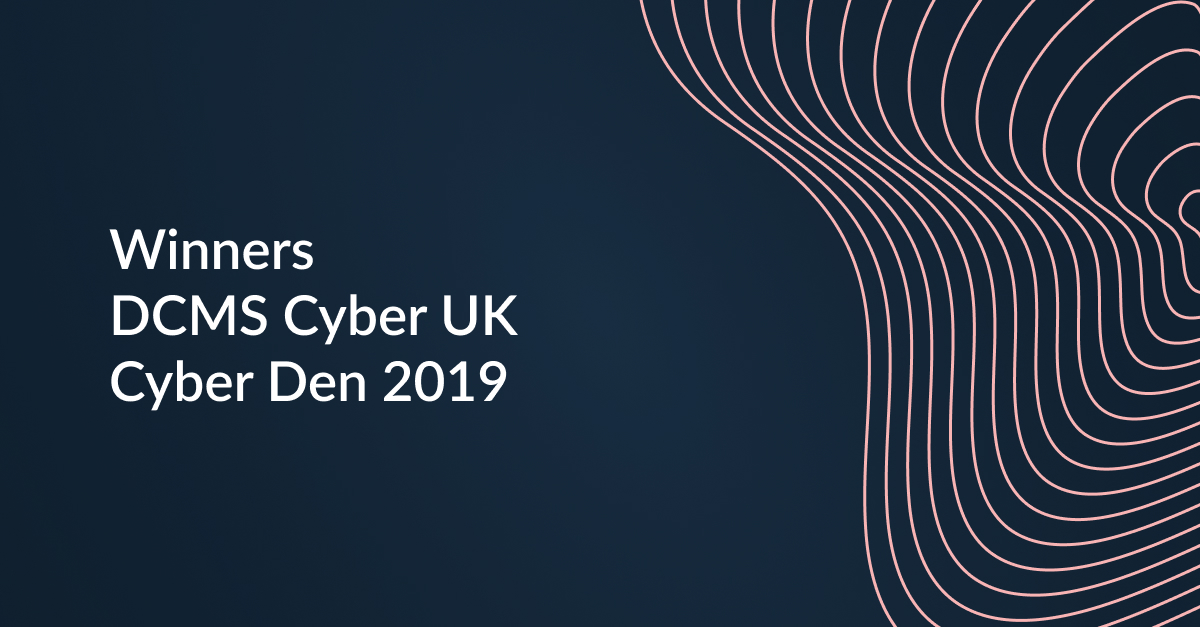Risk LedgerDan & Haydn